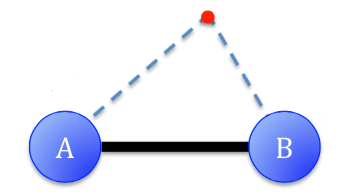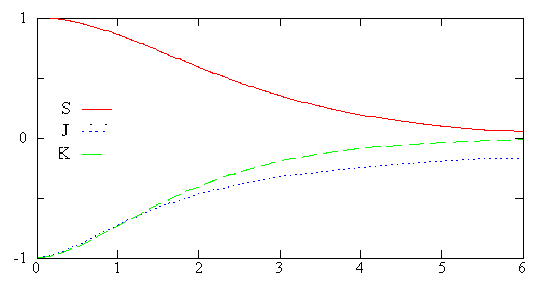Skip to main content

# Worksheet 10: The Dihydrogen Cation

Name: ______________________________

Section: _____________________________

Student ID#:__________________________

Work in groups on these problems. You should try to answer the questions without referring to your textbook. If you get stuck, try asking another group for help.

### The Simplest Molecule has a One-Electron Bond

The simplest molecule, $$H_2^+$$ consists of two protons held together by the electrostatic force of a single electron. Clearly the two protons, two positive charges, repeal each other. The protons must be held together by an attractive Coulomb force that opposes the repulsive Coulomb force. A negative charge density between the two protons would produce the required counter-acting Coulomb force needed to pull the protons together. So intuitively, to create a chemical bond between two protons or two positively charged nuclei, a high density of negative charge between them is needed.### Q1

Using atomic units - Meaning the constants are defined as $$\hbar = 1$$, $$m_e = 1$$, $$e = 1$$ and $$\frac{1}{4\pi\epsilon_0} = 1$$, what is the kinetic energy of the electron in the $$H_2^+$$ Hamiltonian, $$\hat T$$?

### Q2

What is the potential energy of the electron interacting with nucleus $$A$$ of the Hamiltonian?

### Q3

What is the potential energy of the electron interacting with nucleus $$B$$ of the Hamiltonian?

## The Born-Oppenheimer Approximation

Because electrons move so much faster than nuclei, we can assume that on the timescale of electron motion, the nuclei are not moving. This separation of electron and nuclear coordinates is called the Born-Oppenheimer approximation. It means that for each calculation of the energy $$R$$ is a fixed distance, performing a separate energy calculation for each possible seperation distance.

#### Q4

What is the potential energy of nucleus $$A$$ interacting with nucleus $$B$$?

### Q5

Within the Born-Oppenheimer approximation to describe the complete Hamiltonian for the electron, do we need to include kinetic energy of the nuclei (i.e., vibrational and rotational motions)? Justify your answer.

### Q6

Do we need to include the potential energy for the nuclei? The potential $$\dfrac {e^2}{4 \pi \epsilon _oR }$$ between the two protons is graphed below:Distance between protons ($$R$$).

### Q7

If we want to use variation method to model the $$H_2^+$$ molecule, what trial wavefunction do you think would be reasonable to guess for the $$H_2^+$$ molecule? Why do we use the variation method when $$H_2^+$$ is exactly solvable (at least within the Born-Oppenheimer approximation)?

## Optimize the Variational Energy to get the Approximate Wavefunction

Using the following function (which is a Linear Combintation of Atomic Orbitals - LCAO - approximation)

$\phi_{trial}=c_1\psi_{1s_A}+c_2\psi_{1s_B}=c_1|1s_A\rangle+c_2|1s_B\rangle$

as a trial wavefunction. The energy of this wavefunction, via the linear variational method and the Hamiltonian, is given by the variational energy:

$E_\phi=\dfrac{\langle\phi|\hat {H}|\phi\rangle}{\langle\phi|\phi\rangle} \label{varE}$

### Q8

Substituting the trial wavefunction into the denominator of the variational energy (Equation \ref{varE}) leads to

$\langle\phi|\phi\rangle=\langle c_11s_A+c_21s_B|c_11s_A+c_21s_B\rangle$

By multiplying the terms in the integral, what are the four terms that arise and their origin?

### Q9

The first integral in the sum is $$\langle c_11s_A|c_11s_A\rangle$$. What is the value of this integral? Why? What about $$\langle c_21s_B|c_21s_B\rangle$$?

### Q10

We also get terms in $$\langle\phi|\phi\rangle$$ that look like $$\langle c_11s_A|c_21s_B\rangle$$. We call this an overlap integral. Explain (in words)what the integral describes.

### Q11

The related overlap integral (just missing the constants) is commonly called $$\langle 1s_A|1s_B\rangle=S$$. Use your expression for $$\langle c_11s_A|c_11s_A\rangle$$ and the definition $$\langle 1s_A|1s_B\rangle=S$$ to write a simple form for $$\langle\phi|\phi\rangle$$

### Q12

The Figure below plots the overlap integral (solid curve) as a function of internuclear distance. Based on this graph, what happens to the overlap integral as the internuclear bond stretches?R

### Q13

The numerator for variational energy (Equation \ref{varE}) is $$\langle\phi|\hat H|\phi\rangle$$. Substituting the trial wavefunction into the numerator yields

$\langle\phi|\hat H|\phi\rangle =\langle c_11s_A+c_21s_B|\hat H|c_11s_A+c_21s_B\rangle.\label{num}$

What four terms arise from this integral?

### Q14

The first term in the expanding Equation \ref{num} is $$\langle c_11s_A|\hat H|c_11s_A\rangle$$. Substitute the Hamiltonian into this expression. How can you simplify this term by rewriting the Hamiltonian into a hydrogen like part and the additional segments caused by the other nucleus?

### Q15

The second term in expanding Equation \ref{num} is $$\langle c_11s_A|\hat H|c_21s_B\rangle$$. Substitute the Hamiltonian into this expression. How can you simplify this term using the same concepts?

### Q16

We define the Coulomb integral as $$J= \langle 1s_A|\dfrac{1}{r_B}|1s_A\rangle$$ and it is graphed above (dotted line). What does that expression mean in words?

### Q16

We define the exchange integral as $$K= \langle 1s_A|\dfrac{1}{r_A}|1s_B\rangle$$and it is graphed above (dashed line). What does that expression mean in words?

### Q17

Using these expressions, what is the total expression for $$\langle\phi|\hat H|\phi\rangle$$?

### Q18

Graph the Total Energy of $$H_2^+$$.

• Was this article helpful?